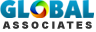# Measure Fixed-end Moments (FEM) & Bending Moment with Fixed Beam Bending Moment CalculatorFixed Beam Bending Moment Calculator is a free online calculator that can be applied to estimate Fixed-end Moments (FEM), Bending Moment as well as Shear Force at any portion of fixed-ended beam on the basis of point load, evenly allocated load, varying load and applied moments.

Guidelines for applying this construction calculator:-

i) Sign convention

• The loads that function upwardly are recognized as positive whereas the loads function downwardly are considered as negative. Sagging bending moment is assumed as positive and hogging as negative. If rotation & moments direct clock-wise then these are called negative whereas rotation in anti-clockwise is considered as negative.
• For fixed-end moments, the anticlock-wise moment toward left-end support is treated as negative; this will induce hogging moments in the beam, while the clock-wise moment toward right-end support is considered as positive that may originate hogging.

ii) All the distances are estimated through reference to the origin of axes that is assumed at the utmost left end of the beam. All distances are recorded in meter and load in kN.

iii) The B.M. values can be instantly obtained at various sections of the beam, by modifying the position of section "x".

iv) To deal with more than one load, consider the sum of the results concerning all the cases (use principle of superposition). In order to plot the bending moment diagram, computed values are applied.

v) This construction calculator is also useful for computing the ordinates of influence line diagram for structures.

# Takeoff Tools